• UA STAT687 线性模型II 最小二乘理论1 普通最小二乘法参数的OLS估计可估函数与Gauss-Markov定理方差的OLS估计正态线性模型与UMVUE Legendre与Gauss在19世纪初提出了最小二乘的思想，1900年Markov证明了最小二乘估计...
UA STAT687 线性模型II 最小二乘理论1 普通最小二乘法参数的OLS估计可估函数与Gauss-Markov定理方差的OLS估计正态线性模型与UMVUE
Legendre与Gauss在19世纪初提出了最小二乘的思想，1900年Markov证明了最小二乘估计的性质良好，在此之后最小二乘就开始广泛应用于线性模型的估计了。对于线性模型
$y=X\beta + \epsilon,E\epsilon=0,Cov(\epsilon)=\sigma^2I$
其中$y,\epsilon$为$n\times 1$的向量，$X$为$n \times p$的Design Matrix，如果$rank(X)\ge p$，称这个线性模型为满秩的；否则称之为降秩的。这部分我们将介绍普通最小二乘法（OLS）、带约束的最小二乘法、广义最小二乘法（GLS）、稳健性、两步法、最小二乘法的几何解释以及常用数值算法，这一篇介绍OLS。
参数的OLS估计
OLS的思路是
$\min_{\beta}\ \ Q = \left\| e \right\|^2 = (y-X\beta)'(y-X\beta)=y'y-2y'X\beta+\beta'X'X\beta$
计算$Q$关于$\beta$的梯度
$\nabla_{\beta} Q=-2X'y+2X'X\beta=0 \Rightarrow X'X\beta = X'y$
这个方程叫做OLS的正则方程，求解这个方程可以得到系数的OLS估计，并且基于这个方程还可以获得残差的性质。$X'y$在$X'$的列空间中，因此这个方程是相容的，可以用系数矩阵的广义逆表示解：
$\hat{\beta} = (X'X)^{-}X'y$
计算$Q$关于$\beta$的Hessian矩阵，
$H_{\beta}Q = 2X'X\ge 0$
因此$\hat{\beta}$使$Q$取最小值，并且最小值点唯一。
下面考虑广义逆的确定。假设$rank(X)\ge p$，则$X'X$是满秩的方阵，
$\hat{\beta} = (X'X)^{-1}X'y$
假设$rank(X)，则$X'X$降秩，它的逆不存在，此时不存在$\beta$的线性无偏估计。
证明
假设$Ay$是线性无偏估计，则$E(Ay) = AX\beta = \beta \Rightarrow AX = I_p \Rightarrow rank(AX)=p$，然而$rank(AX)\le rank(X)，这就出现了矛盾。
可估函数与Gauss-Markov定理
如果我们就停留在上一节的讨论，那么OLS的局限性是很强的，因为我们有时并不一定是要关注$\beta$，也不一定总是需要线性无偏估计。
在$rank(X)这种情况中，称$\beta$是不可估计，但我们可以考察$\beta$的某种线性组合（参考RCD的线性组合与contract理论）$c'\beta$，如果$\exists a$，$n \times 1$的列向量，使得$E[a'y]=c'\beta$，就称$c'\beta$是可估函数。显然$c$属于$X'$的列空间。如果$c_1'\beta$与$c_2'\beta$中的$c_1,c_2$线性无关，则称$c_1'\beta$与$c_2'\beta$线性无关。因为$c$属于$X'$的列空间，所以一组线性无关的可估函数最多有$rank(X)$个，并且据此可以得出$\exists a$, $c=X'a$，进而
$c'\hat{\beta} = c'(X'X)^{-}X'y=a'X(X'X)^{-}X'y$
参考矩阵分析与多元统计那个系列，包含广义逆的$X(X'X)^{-}X'$一项与广义逆的选取无关，这个性质保证$c'\hat{\beta}$是一个良定义。另外，
$E(c'\hat{\beta}) = a'X(X'X)^{-}X'Ey=a'X(X'X)^{-}X'X\beta=a'X\beta=c'\beta$
说明$c'\hat{\beta}$是可估函数$c\beta$的无偏估计。基于这些分析，我们可以自信地定义$c'\hat{\beta}$为$c'\beta$的OLS估计。OLS是具有唯一性的，但在回归那个系列我们讨论过，线性无偏估计不具有唯一性，但Gauss-Markov定理指出OLS估计是最优线性无偏估计（Best Linear Unbiased Estimator，BLUE）：
Gauss-Markov定理 $c'\hat{\beta}$是$c'\beta$的BLUE。
证明 无偏性说明过了，下面讨论最优性（所有线性无偏估计中方差最小）。计算$c'\hat{\beta}$的方差：
$Var(c'\hat{\beta}) = Var(a'X(X'X)^{-}X'y) =\sigma^2a'X(X'X)^{-}X'X(X'X)^{-}X'a \\= \sigma^2a'X(X'X)^{-}X'a = \sigma^2 c'(X'X)^{-}c$
假设$b'y$是$c'\beta$的另一个线性无偏估计，则$E(b'y)=b'X\beta=c'\beta$，也就是$c=X'b$，计算
$Var(b'y) = \sigma^2b'b$
考虑两个方差的差，
$Var(b'y)-Var(c'\hat{\beta}) =\sigma^2[b'b-c'(X'X)^{-}c] \\ = \sigma^2[b'-c'(X'X)^{-}][b-(X'X)^{-}c]=\sigma^2 \left\| b-(X'X)^{-}c\right\| \ge 0$
所以OLS是BLUE。
方差的OLS估计
模型的残差为$e=y-X\hat{\beta}=(I-P_X)y$，其中$P_X=X(X'X)^{-}X'$是到$X$列空间中的投影矩阵，可以计算
$E\hat{e}=(I-P_X)X\beta=X\beta - X(X'X)^{-}X'X\beta=0 \\ Cov(\hat{e})=\sigma^2(I-P_X)(I-P_X)'=\sigma^2(I-P_X)$
基于这个结果可以构造$\sigma^2$的无偏估计为
$\hat\sigma^2=\frac{\hat{e}'\hat{e}}{n-rank(X)}$
证明
首先$\hat{e}'\hat{e}=y'(I-P_X)y$，下面计算
$E[\hat{e}'\hat{e}]=(X\beta)'(I-P_X)+tr[(I-P_X)Cov(y)] \\=\sigma^2tr(I-P_X)=\sigma^2(n-rank(X))$
第一行到第二行应用的第一个结论是$(I-P_X)X=0$，用到的第二个结论是如果$EX=\mu,Cov(X)=\Sigma$，则
$E[X'AX]=\mu'A\mu+tr(A\Sigma)$
下面证明这个恒等式。计算
$X'AX=(X-\mu+\mu)'A(X-\mu+\mu) \\ = (X-\mu)'A(X-\mu)+\mu'A(X-\mu)+(X-\mu)'A\mu+\mu'A\mu$
接下来求期望，
$E[\mu'A(X-\mu)]=E[\mu'AX]-\mu'A\mu=0$
类似的，第三项的期望也为0，计算第一项的期望，
$E[(X-\mu)'A(X-\mu)]=Etr[(X-\mu)'A(X-\mu)] \\ = Etr[A(X-\mu)(X-\mu)'] = trAE[(X-\mu)(X-\mu)'] = tr(A\Sigma)$
这就完成了整个证明。在回归那个系列给出过另一种证明，但思路类似，都是把数量用trace表示，在利用trace中矩阵乘法满足交换律的技巧。
正态线性模型与UMVUE
需要注意的是OLS对随机误差的分布形式是没有要求的，只有当我们试图对OLS估计量做统计推断的时候，我们才需要考虑随机误差的分布形式。这是OLS与MLE一个很大的区别，MLE为了获得估计量就需要在一开始引入某种特定的分布，再去最大化给定数据在这种分布下的似然。
现在假设随机误差服从正态分布，则OLS有一些优越的性质，这些性质在MATH 564、566、571A的博客中都有过证明了，所以这里就简单归纳一下：

OLS也是MLE，且$c'\hat{\beta} \sim N(c'\beta,\sigma^2c'(X'X)^{-}c)$
$\sigma^2$的MLE是$\hat{e}'\hat{e}/n$，且$(n-rank(X))\hat{\sigma}^2\sim \sigma^2 \chi^2_{n-rank(X)}$
$c'\hat{\beta}$与$\hat{\sigma}^2$互相独立
$c'\hat{\beta}$是唯一的UMVUE


展开全文统计学 线性模型 机器学习
• 普通最小二乘法（OLS）（有时也称为线性最小二乘法）估计线性回归线的参数，从而使样本点垂直距离（残差或误差）之和最小化。 from sklearn.preprocessing import StandardScaler import numpy as np import ...
用普通最小二乘法（OLS）（有时也称为线性最小二乘法）估计线性回归线的参数，从而使样本点的垂直距离（残差或误差）之和最小化。
from sklearn.preprocessing import StandardScaler
import numpy as np
import pandas as pd
import matplotlib.pyplot as plt

df = pd.read_csv('xxx\\housing.data.txt',
header=None,
sep='\s+')

df.columns = ['CRIM', 'ZN', 'INDUS', 'CHAS',
'NOX', 'RM', 'AGE', 'DIS', 'RAD',
'TAX', 'PTRATIO', 'B', 'LSTAT', 'MEDV']
print(df.head())

class LinearRegressionGD(object):

def __init__(self, eta=0.001, n_iter=20):
self.eta = eta
self.n_iter = n_iter

def fit(self, X, y):
self.w_ = np.zeros(1 + X.shape)
self.cost_ = []

for i in range(self.n_iter):
output = self.net_input(X)
errors = (y - output)
self.w_[1:] += self.eta * X.T.dot(errors)
self.w_ += self.eta * errors.sum()
cost = (errors**2).sum() / 2.0
self.cost_.append(cost)
return self

def net_input(self, X):
return np.dot(X, self.w_[1:]) + self.w_

def predict(self, X):
return self.net_input(X)

X = df[['RM']].values
y = df['MEDV'].values

sc_x = StandardScaler()
sc_y = StandardScaler()
X_std = sc_x.fit_transform(X)
# scikit-learn 的大多数转换器期望数据存储在二维阵列
y_std = sc_y.fit_transform(y[:, np.newaxis]).flatten()

lr = LinearRegressionGD()
lr.fit(X_std, y_std)

# 当使用像梯度下降的优化算法时，以训练集迭代次数作为成本函数绘制成本图,
# 来检查算法是否收敛到了最低成本
#（这里指全局性 最小成本值）确实是个不错的主意
plt.plot(range(1, lr.n_iter+1), lr.cost_)
plt.ylabel('SSE')
plt.xlabel('Epoch')
#plt.tight_layout()
#plt.savefig('images/10_05.png', dpi=300)
plt.show()

# 观察线性回归与训练数据的吻合程度
def lin_regplot(X, y, model):
# s：指定散点图点的大小，默认为20，通过传入新的变量，实现气泡图的绘制
# c：指定散点图点的颜色，默认为蓝色
# edgecolors：设置散点边界线的颜色
plt.scatter(X, y, c='steelblue', edgecolor='white', s=70)
plt.plot(X, model.predict(X), color='black', lw=2)
return

lin_regplot(X_std, y_std, lr)
plt.xlabel('Average number of rooms [RM] (standardized)')
plt.ylabel('Price in $1000s [MEDV] (standardized)') #plt.savefig('images/10_06.png', dpi=300) plt.show() # 调用StandardScaler的inverse_transform方法， # 把价格的预测结果恢复到以1000美元为单位的坐标轴 num_rooms_std = sc_x.transform(np.array([[5.0]])) # 有五个房间房屋的价格 price_std = lr.predict(num_rooms_std) print("Price in$1000s: %.3f" % sc_y.inverse_transform(price_std))

# 值得一提的是如果处理标准化变量，从技术角度来说，不需要更新截距的权重，
# 因为在这些情况下，y轴的截距总是0。可以通过打印权 重来快速确认这一点
print('Slope: %.3f' % lr.w_)
print('Intercept: %.3f' % lr.w_)

运行结果：
CRIM    ZN  INDUS  CHAS    NOX  …    TAX  PTRATIO       B  LSTAT  MEDV
0  0.00632  18.0   2.31     0  0.538  …  296.0     15.3  396.90   4.98  24.0
1  0.02731   0.0   7.07     0  0.469  …  242.0     17.8  396.90   9.14  21.6
2  0.02729   0.0   7.07     0  0.469  …  242.0     17.8  392.83   4.03  34.7
3  0.03237   0.0   2.18     0  0.458  …  222.0     18.7  394.63   2.94  33.4
4  0.06905   0.0   2.18     0  0.458  …  222.0     18.7  396.90   5.33  36.2
[5 rows x 14 columns]
Price in $1000s: 10.840 Slope: 0.695 Intercept: -0.000 运行结果图：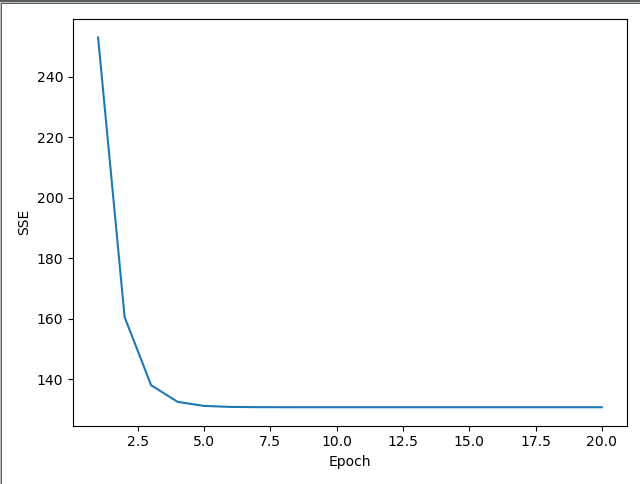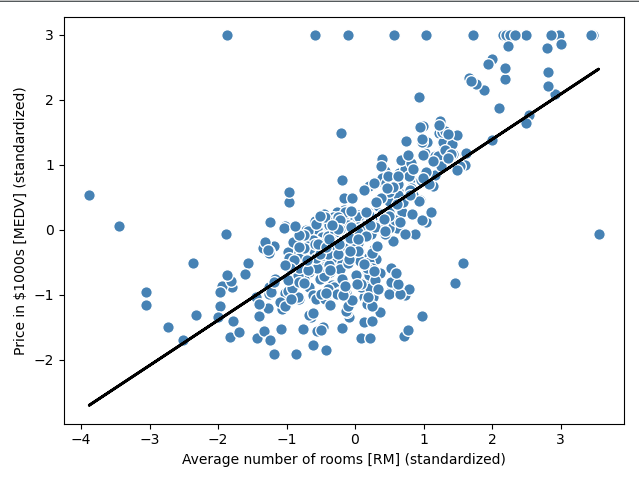展开全文算法 python 机器学习 • 2.2 最小二乘估计方法 2.3 模型效果分析 2.4 显著性检验 2.5 变量筛选方法 2.6 多重相关性问题 第3章 数据表成分提取方法 3.1 表内成分提取——主成分分析 3.2 表间成分提取——典型相关... • 目的是估计参数β。 回想一下，针对该概率使用该函数是 （对数）似然函数 对数似然 其中。数值方法基于（数值）下降梯度来计算似然函数最大值。对数似然（负）是以下函数 negLogLik = function... 原文链接：http://tecdat.cn/?p=21379 本文我们对逻辑回归和样条曲线进行介绍。 logistic回归基于以下假设：给定协变量x，Y具有伯努利分布，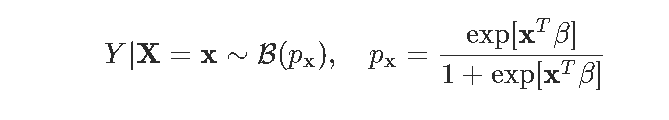目的是估计参数β。 回想一下，针对该概率使用该函数是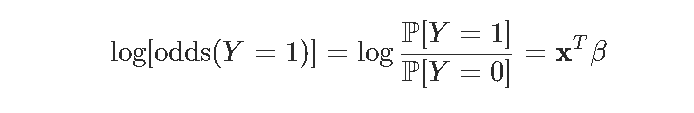（对数）似然函数 对数似然其中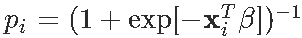。数值方法基于（数值）下降梯度来计算似然函数的 最大值。对数似然（负）是以下函数  negLogLik = function(beta){ -sum(-y*log(1 + exp(-(X%*%beta))) - (1-y)*log(1 + exp(X%*%beta))) } 现在，我们需要一个起始点来启动算法 optim(par = beta_init, negLogLik, hessian=TRUE, method = "BFGS", control=list(abstol=1e-9)) 在这里，我们得到  logistic_opt$par
(Intercept)        FRCAR        INCAR        INSYS
1.656926397  0.045234029 -2.119441743  0.204023835
PRDIA        PAPUL        PVENT        REPUL
-0.102420095  0.165823647 -0.081047525 -0.005992238

让我们在这里验证该输出是否有效。例如，如果我们（随机）更改起点的值会怎么样


plot(v_beta)
par(mfrow=c(1,2))
hist(v_beta[,1],xlab=names( )[ ])
hist(v_beta[,2],xlab=names( ))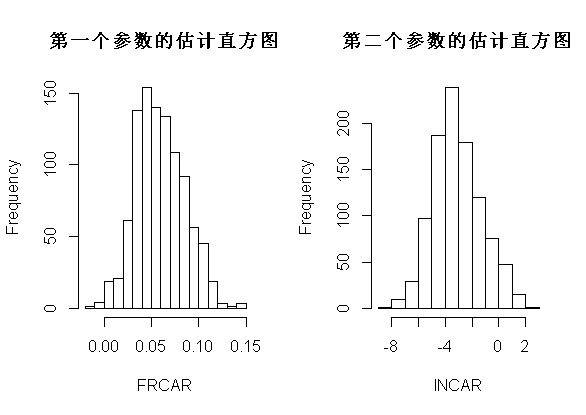这里有个问题。注意，我们不能在这里进行数值优化。我们可以考虑使用其他优化方法


logLikelihoodLogitStable = function(vBeta, mX, vY) {
-sum(vY*(mX %*% vBeta - log(1+exp(mX %*% vBeta) +
(1-vY)*(-log(1 + exp(mX %*% vBeta))

optimLogitLBFGS = optimx(beta_init, logLikelihoodLogitStable,


最优点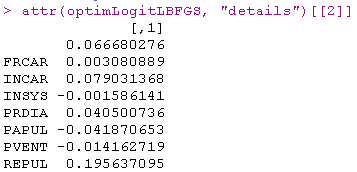结果不理想。

我们使用的技术基于以下思想，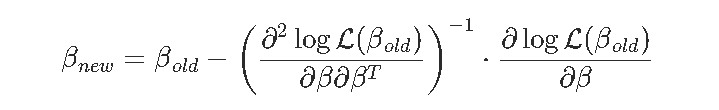问题是我的计算机不知道一阶和二阶导数。

可以使用这种计算的函数


logit = function(x){1/(1+exp(-x))}

for(i in 1:num_iter){
grad = (t(X)%*%(logit(X%*%beta) - y))
beta = beta - ginv(H)%*%grad
LL[i] = logLik(beta, X, y)


以我们的OLS起点，我们获得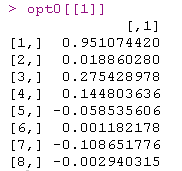如果我们尝试另一个起点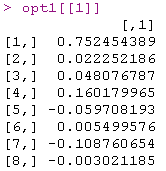一些系数非常接近。然后我们尝试其他方法。

牛顿（或费舍尔）算法

在计量经济学教科书里，您可以看到：beta=as.matrix(lm(Y~0+X)$coefficients for(s in 1:9){ pi=exp(X%*%beta[,s])/(1+exp(X%*%beta[,s])) gradient=t(X)%*%(Y-pi) omega=matrix(0,nrow(X),nrow(X));diag(omega)=(pi*(1-pi))  在这里观察到，我仅使用该算法的十次迭代。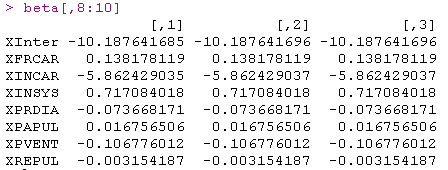事实是，收敛似乎非常快。而且它相当鲁棒，看看我们改变起点会得到什么 beta=as.matrix(lm(Y~0+X)$coefficients,ncol=1)*runif(8)
for(s in 1:9){
pi=exp(X%*%beta[,s])/(1+exp(X%*%beta[,s]))
gradient=t(X)%*%(Y-pi)
omega=matrix(0,nrow(X),nrow(X));diag(omega)=(pi*(1-pi))
Hessian=-t(X)%*%omega%*%X
beta=cbind(beta,beta[,s]-solve(Hessian)%*%gradient)}
beta[,8:10]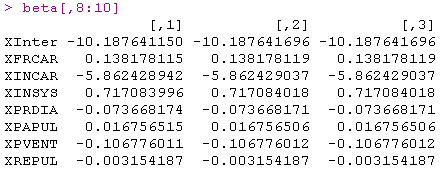效果提高了，并且可以使用矩阵的逆获得标准偏差。

标准最小二乘

我们更进一步。我们已经看到想要计算类似但是实际，这是一个标准的最小二乘问题这里唯一的问题是权重Δold是未知β的函数。但是实际上，如果我们继续迭代，我们应该能够解决它：给定β，我们得到了权重，并且有了权重，我们可以使用加权的OLS来获取更新的β。这就是迭代最小二乘的想法。

该算法


beta_init = lm(PRONO~.,data=df)\$coefficients

for(s in 1:1000){
omega = diag(nrow(df))
diag(omega) = (p*(1-p))


输出在这里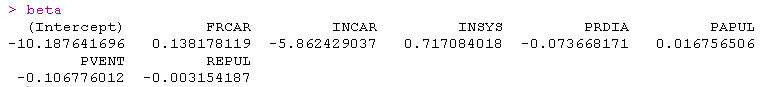结果很好，我们在这里也有估计量的标准差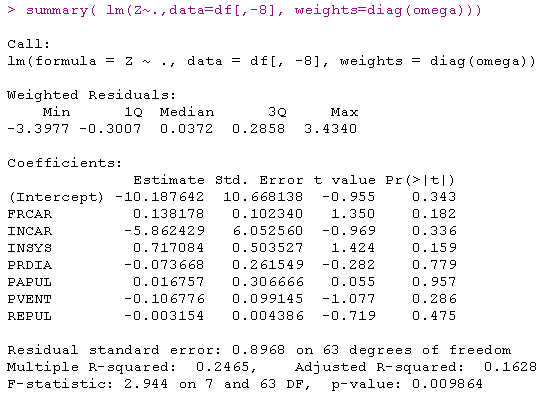标准逻辑回归glm函数：

当然，可以使用R内置函数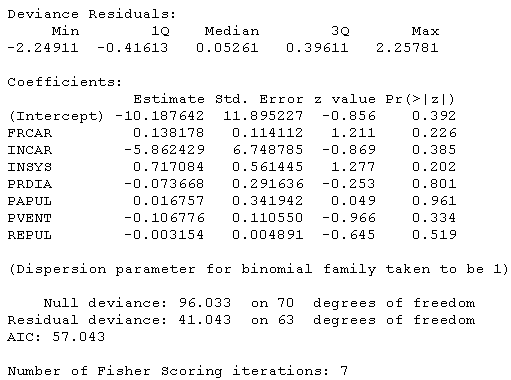可视化

让我们在第二个数据集上可视化从逻辑回归获得的预测


image(u,u,v ,breaks=(0:10)/10)
points(x,y,pch=19 )
points(x,y,pch=c(1,19)
contour(u,u,v,levels = .5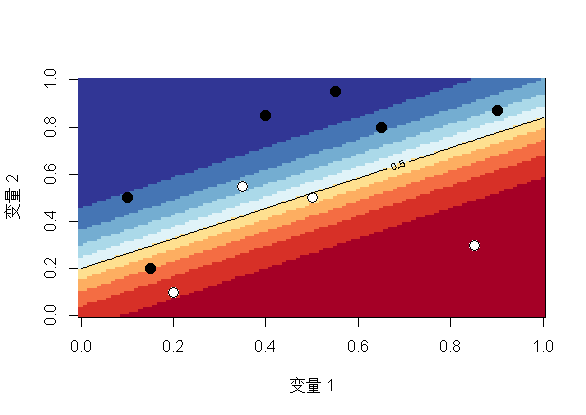这里的水平曲线-或等概率-是线性的，因此该空间被一条直线（或更高维的超平面）一分为二（0和1，生存和死亡，白色和黑色）此外，由于我们是线性模型，因此，如果更改截距（为创建两个类别的阈值），我们将获得平行的另一条直线（或超平面）。

接下来，我们将约会样条曲线以平滑那些连续的协变量。

分段线性样条函数

我们从“简单”回归开始（只有一个解释变量），我们可以想到的最简单的模型来扩展我们上面的线性模型， 是考虑一个分段线性函数，它分为两部分。最方便的方法是使用正部函数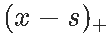（如果该差为正，则为x和s之间的差，否则为0）。如是以下连续的分段线性函数，在s处划分。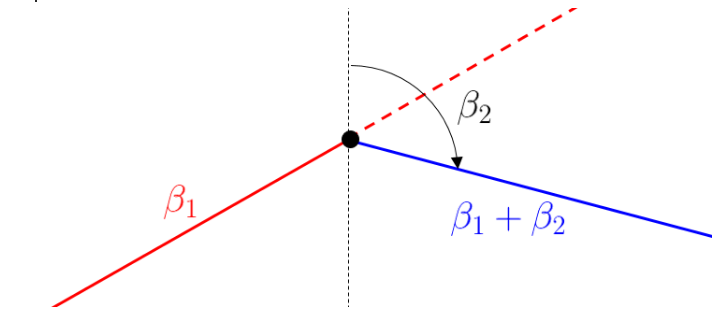对于较小的x值，线性增加，斜率β1；对于较大的x值，线性减少。因此，β2被解释为斜率的变化。

当然，可以考虑多个结。获得正值的函数如下

pos = function(x,s) (x-s)*(x<=s)

然后我们可以在回归模型中直接使用它

回归的输出在这里



Coefficients:
Estimate Std. Error z value Pr(&gt;|z|)
(Intercept)     -0.1109     3.2783  -0.034   0.9730
INSYS           -0.1751     0.2526  -0.693   0.4883
pos(INSYS, 15)   0.7900     0.3745   2.109   0.0349 *
pos(INSYS, 25)  -0.5797     0.2903  -1.997   0.0458 *

因此，对于很小的值，原始斜率并不重要，但是在15以上时，它会变得明显为正。而在25以上，又发生了重大变化。我们可以对其进行绘图以查看发生了什么


plot(u,v,type="l")
points(INSYS,PRONO)
abline(v=c(5,15,25,55)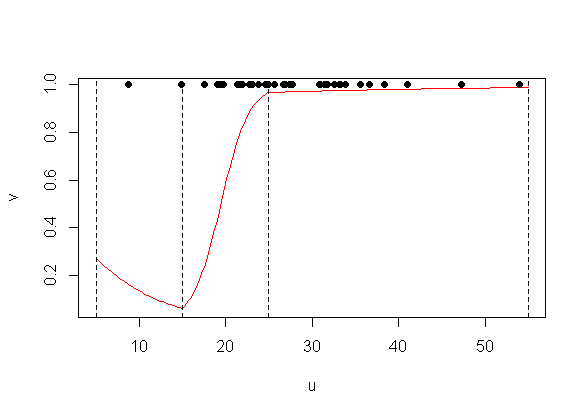使用bs（）线性样条曲线

使用GAM模型，情况略有不同。我们将在这里使用所谓的 b样条曲线，

我们可以用边界结点（5,55）和结 {15,25}定义样条函数


B = bs(x,knots=c(15,25),Boundary.knots=c(5,55),degre=1)
matplot(x,B,type="l",lty=1,lwd=2,col=clr6)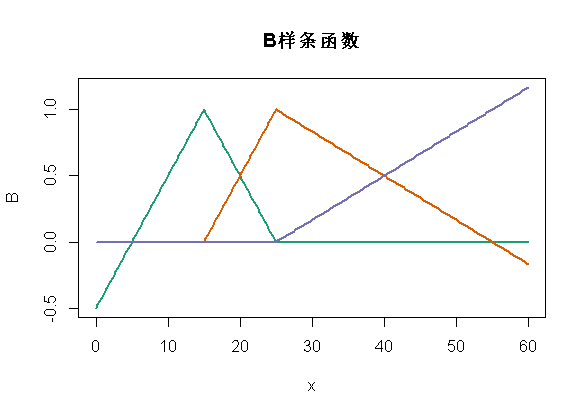如我们所见，此处定义的函数与之前的函数不同，但是在每个段（5,15）（15,25）和（25,55）。但是这些函数（两组函数）的线性组合将生成相同的空间。换个角度说，对输出的解释会不同，预测应该是一样的。



Coefficients:
Estimate Std. Error z value Pr(&gt;|z|)
(Intercept)    -0.9863     2.0555  -0.480   0.6314
bs(INSYS,..)1  -1.7507     2.5262  -0.693   0.4883
bs(INSYS,..)2   4.3989     2.0619   2.133   0.0329 *
bs(INSYS,..)3   5.4572     5.4146   1.008   0.3135

观察到像以前一样存在三个系数，但是这里的解释更加复杂了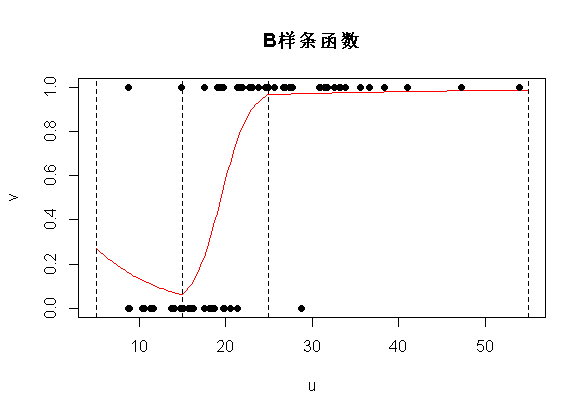但是，预测结果很好。

分段二次样条

让我们再往前走一步...我们是否也可以具有导数的连续性？考虑抛物线函数，不要对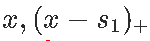和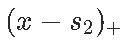进行分解，考虑对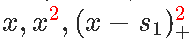和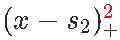进行分解。

Coefficients:
Estimate Std. Error z value Pr(&gt;|z|)
(Intercept)      29.9842    15.2368   1.968   0.0491 *
poly(INSYS, 2)1 408.7851   202.4194   2.019   0.0434 *
poly(INSYS, 2)2 199.1628   101.5892   1.960   0.0499 *
pos2(INSYS, 15)  -0.2281     0.1264  -1.805   0.0712 .
pos2(INSYS, 25)   0.0439     0.0805   0.545   0.5855

不出所料，这里有五个系数：截距和抛物线函数的三个参数，然后是中间两个附加项–此处（15,25）–以及右侧的部分。当然，对于每个部分，只有一个自由度，因为我们有一个抛物线函数（三个系数），但是有两个约束（连续性和一阶导数的连续性）。

在图上，我们得到以下内容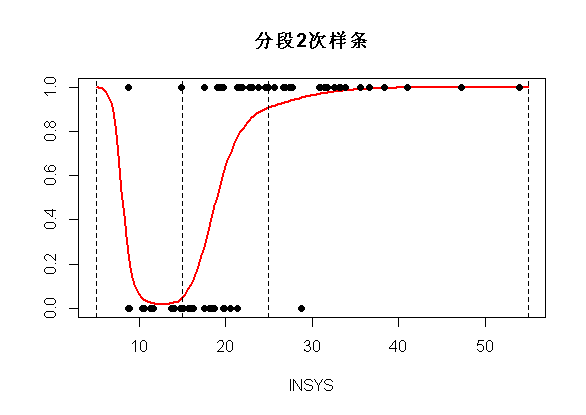使用bs（）二次样条

当然，我们可以使用R函数执行相同的操作。但是和以前一样，这里的函数有所不同


matplot(x,B,type="l",col=clr6)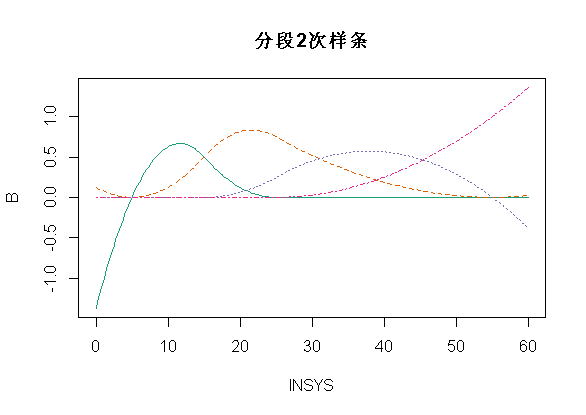如果我们运行R代码，得到

 glm(y~bs(INSYS knots=c(15,25),
Boundary.knots=c(5,55),degre=2)

Coefficients:
Estimate Std. Error z value Pr(&gt;|z|)
(Intercept)       7.186      5.261   1.366   0.1720
bs(INSYS, ..)1  -14.656      7.923  -1.850   0.0643 .
bs(INSYS, ..)2   -5.692      4.638  -1.227   0.2198
bs(INSYS, ..)3   -2.454      8.780  -0.279   0.7799
bs(INSYS, ..)4    6.429     41.675   0.154   0.8774

预测是完全相同的


plot(u,v,ylim=0:1,type="l",col="red")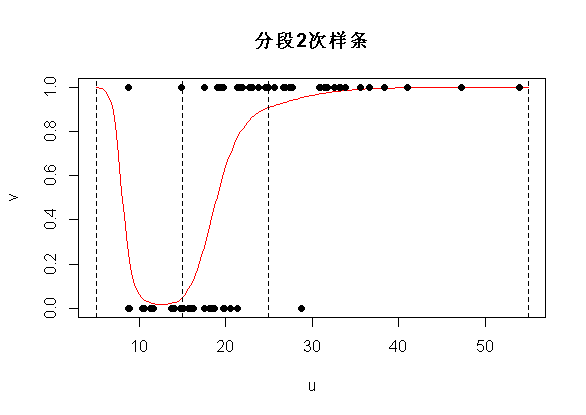三次样条

我们可以使用三次样条曲线。我们将考虑对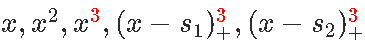进行分解，得到时间连续性，以及前两个导数的连续性。如果我们使用bs函数，则如下

matplot(x,B,type="l",lwd=2,col=clr6,lty=1
abline(v=c(5,15,25,55),lty=2)现在的预测将是

bs(x,knots=c(15,25),
Boundary.knots=c(5,55),degre=3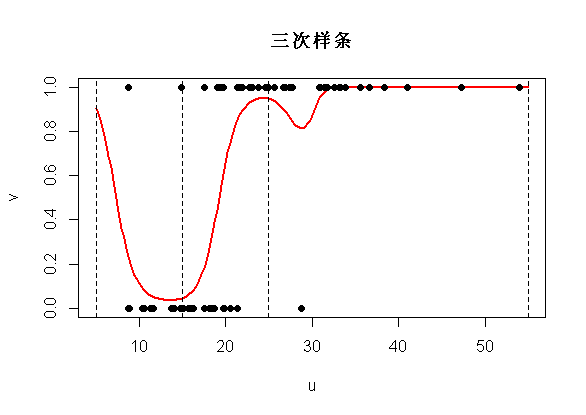结的位置

在许多应用程序中，我们不想指定结的位置。我们只想说（三个）中间结。可以使用

bs(x,degree=1,df=4)

可以查看


bs(x, degree = 1L, knots = c(15.8, 21.4, 27.15),
Boundary.knots = c(8.7, 54), intercept = FALSE)

它为我们提供了边界结的位置（样本中的最小值和最大值），也为我们提供了三个中间结。观察到实际上，这五个值只是（经验）分位数

quantile( ,(0:4)/4)
0%   25%   50%   75%  100%
8.70 15.80 21.40 27.15 54.00

如果我们绘制预测，我们得到

 plot(u,v,ylim=0:1,type="l",col="red",lwd=2)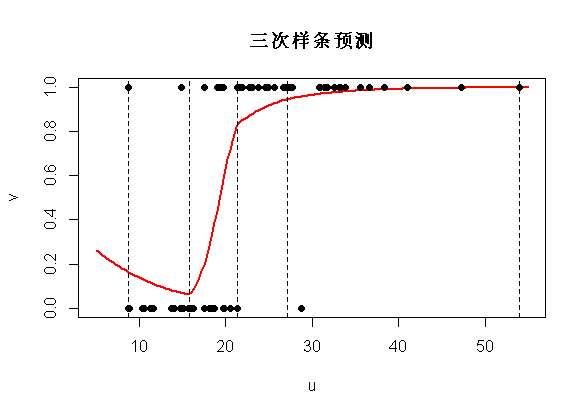如果我们回到logit变换之前的计算，我们清楚地看到断点是不同的分位数


plot(x,y,type="l",col="red",lwd=2)
abline(v=quantile(my ,(0:4)/4),lty=2)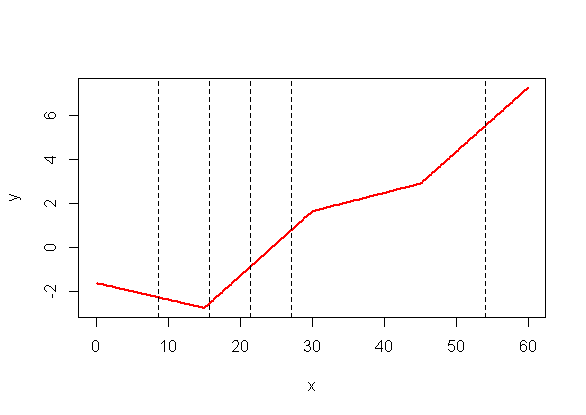如果我们没有指定，则不会得到任何结…


bs(x, degree = 2L, knots = numeric(0),
Boundary.knots = c(8.7,54), intercept = FALSE)

如果我们看一下预测

 predict(reg,newdata=data.frame(u),type="response")


实际上，这和二次多项式回归是一样的（如预期的那样）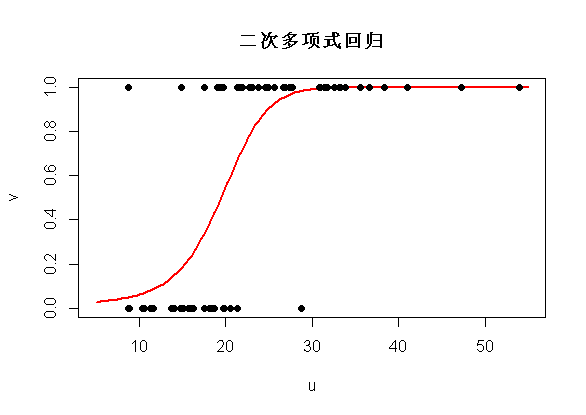相加模型

现在考虑第二个数据集，包含两个变量。这里考虑一个模型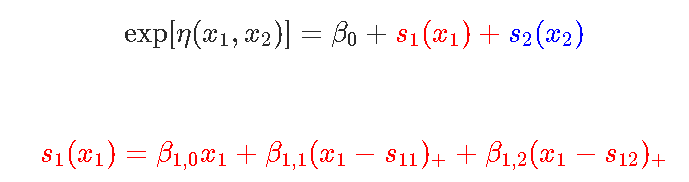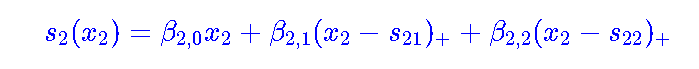然后我们用glm函数来实现相加模型的思想。

glm(y~bs(x1,degree=1,df=3)+bs(x2,degree=1,df=3), family=binomial(link =
v = outer(u,u,p)
image(u,u,v, ",col=clr10,breaks=(0:10)/10)


现在，我们能够得到一个“完美”的模型，所以，结果似乎不再连续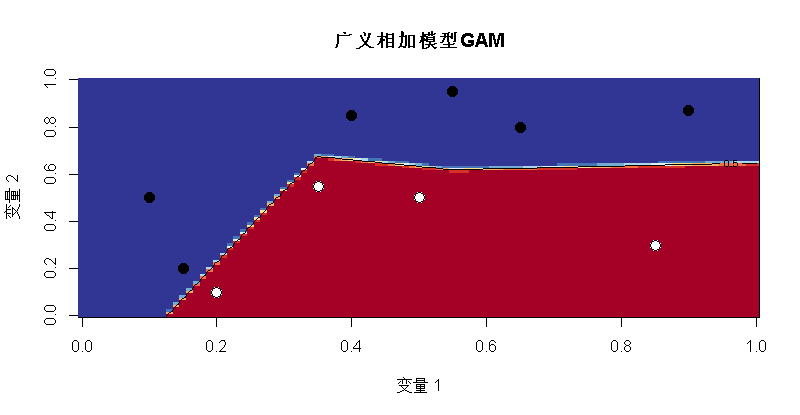persp(u,u,v,theta=20,phi=40,col="green"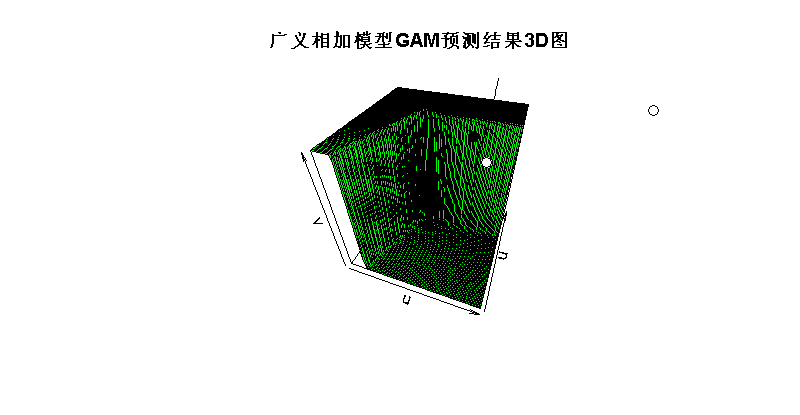当然，它是分段线性的，有超平面，有些几乎是垂直的。
我们也可以考虑分段二次函数



contour(u,u,v,levels = .5,add=TRUE)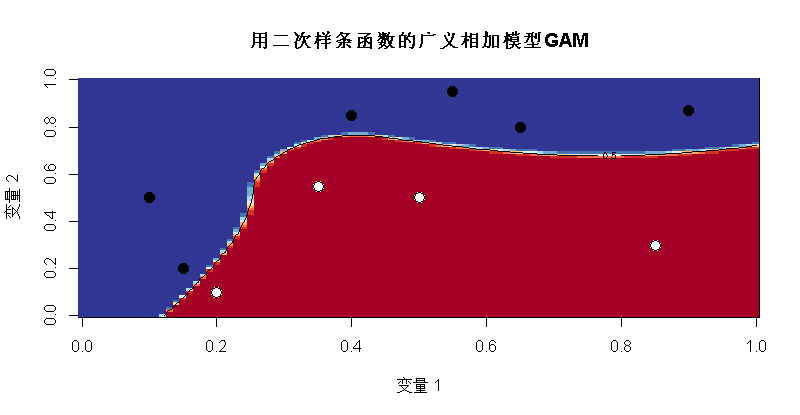有趣的是，我们现在有两个“完美”的模型，白点和黑点的区域不同。

在R中，可以使用mgcv包来运行gam回归。它用于广义相加模型，但这里只有一个变量，所以实际上很难看到“可加”部分，可以参考其他GAM文章。最受欢迎的见解

1.R语言多元Logistic逻辑回归 应用案例

2.面板平滑转移回归(PSTR)分析案例实现

3.matlab中的偏最小二乘回归（PLSR）和主成分回归（PCR）

4.R语言泊松Poisson回归模型分析案例

5.R语言回归中的Hosmer-Lemeshow拟合优度检验

6.r语言中对LASSO回归，Ridge岭回归和Elastic Net模型实现

7.在R语言中实现Logistic逻辑回归

8.python用线性回归预测股票价格

9.R语言如何在生存分析与Cox回归中计算IDI，NRI指标

展开全文R语言 广义相加模型 GAM
• ## 普通最小二乘法的推导证明

万次阅读 多人点赞 2018-01-28 00:09:20
这里的“二乘”指的是用平方来度量观测点与估计点的远近（在古汉语中“平方”称为“二乘”），“最小”指的是参数的估计值要保证各个观测点与估计点的距离的平方和达到最小。从这个上也可以看出，最小二乘也可用于...
最小二乘法

1、什么是最小二乘思想？

​   简单地说，最小二乘的思想就是要使得观测点和估计点的距离的平方和达到最小.这里的“二乘”指的是用平方来度量观测点与估计点的远近（在古汉语中“平方”称为“二乘”），“最小”指的是参数的估计值要保证各个观测点与估计点的距离的平方和达到最小。从这个上也可以看出，最小二乘也可用于拟合数据模型。

2. 最小二乘法推导

​   我们以最简单的一元线性模型来解释最小二乘法。什么是一元线性模型呢？ 监督学习中，如果预测的变量是离散的，我们称其为分类（如决策树，支持向量机等），如果预测的变量是连续的，我们称其为回归。回归分析中，如果只包括一个自变量和一个因变量，且二者的关系可用一条直线近似表示，这种回归分析称为一元线性回归分析。如果回归分析中包括两个或两个以上的自变量，且因变量和自变量之间是线性关系，则称为多元线性回归分析。对于二维空间线性是一条直线；对于三维空间线性是一个平面，对于多维空间线性是一个超平面…

​   对于一元线性回归模型, 假设从总体中获取了 nn<!--//--><![CDATA[//><!--
n
//--><!]]> 组观察值 （X1，Y1）（X1，Y1）<!--//--><![CDATA[//><!--
（X_1，Y_1）
//--><!]]>，（X2，Y2）（X2，Y2）<!--//--><![CDATA[//><!--
（X_2，Y_2）
//--><!]]>，
…，（Xn，Yn）（Xn，Yn）<!--//--><![CDATA[//><!--
（X_n，Y_n）
//--><!]]>。对于平面中的这 nn<!--//--><![CDATA[//><!--
n
//--><!]]> 个点，可以使用无数条曲线来拟合。要求样本回归函数尽可能好地拟合这组值。综合起来看，这条直线处于样本数据的中心位置最合理。
​   选择最佳拟合曲线的标准可以确定为：使总的拟合误差（即总残差）达到最小。有以下三个标准可以选择：

​   （1）用“残差和最小”确定直线位置是一个途径。但很快发现计算“残差和”存在相互抵消的问题。

​   （2）用“残差绝对值和最小”确定直线位置也是一个途径。但绝对值的计算比较麻烦。

​   （3）最小二乘法的原则是以“残差平方和最小”确定直线位置。用最小二乘法除了计算比较方便外，得到的估计量还具有优良特性。这种方法对异常值非常敏感。

​   最常用的是普通最小二乘法（ Ordinary  Least Square，OLS）：所选择的回归模型应该使所有观察值的残差平方和达到最小。

公式推导

​   1 拟合直线：y=a+bxy=a+bx<!--//--><![CDATA[//><!--
y = a + bx
//--><!]]>

​   2 有任意观察点 (xi,yi)(xi,yi)<!--//--><![CDATA[//><!--
(x_i, y_i)
//--><!]]>

​   3 误差为 di=yi−(a+bxi)di=yi−(a+bxi)<!--//--><![CDATA[//><!--
d_i = y_i - (a + bx_i)
//--><!]]>

​   4 当 D=∑ni=1d2i=0D=∑i=1ndi2=0<!--//--><![CDATA[//><!--
D=\sum_{i=1}^n d_i^2 = 0
//--><!]]> 取值最小时，直线拟合度最高。

​   5 D=∑ni=1d2i=∑ni=1(yi−a−bxi)2D=∑i=1ndi2=∑i=1n(yi−a−bxi)2<!--//--><![CDATA[//><!--
D = \sum_{i=1}^n d_i^2 = \sum_{i=1}^n(y_i -a - bx_i)^2
//--><!]]>, 对 a,ba,b<!--//--><![CDATA[//><!--
a, b
//--><!]]> 分别求一阶偏导： ∂D∂a=∑i=1n2(yi−a−bxi)∗(−1)∂D∂a=∑i=1n2(yi−a−bxi)∗(−1)<!--//--><![CDATA[//><!--
\frac{\partial D}{\partial a} = \sum_{i=1}^n2(y_i -a -bx_i)*(-1)
//--><!]]>∂D∂b=2∑i=1n(yi−a−bxi)(−xi)=−2(∑i=1nxiyi−a∑i=1nxi−b∑i=1nx2i)∂D∂b=2∑i=1n(yi−a−bxi)(−xi)=−2(∑i=1nxiyi−a∑i=1nxi−b∑i=1nxi2)<!--//--><![CDATA[//><!--
\frac{\partial D}{\partial b} = 2\sum_{i=1}^n(y_i -a -bx_i)(-x_i) \\
= -2(\sum_{i=1}^n x_iy_i -a\sum_{i=1}^nx_i -b\sum_{i=1}^nx_i^2)
//--><!]]>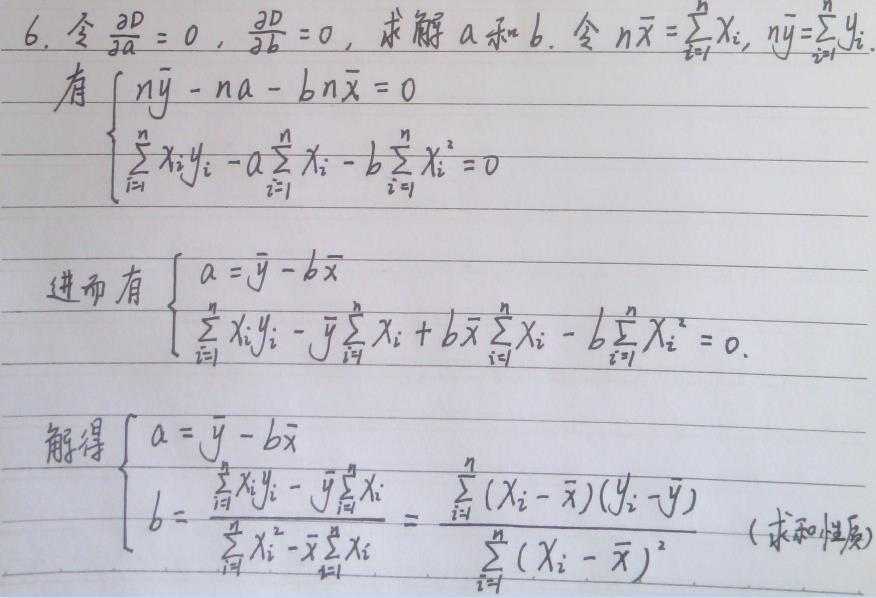求和性质

求和性质，具体可以参考Introductory Econometrics A Modern Approach (Fourth Edition) 一书（计量经济学导论，第4版，杰弗里·M·伍德里奇 著）的附录A。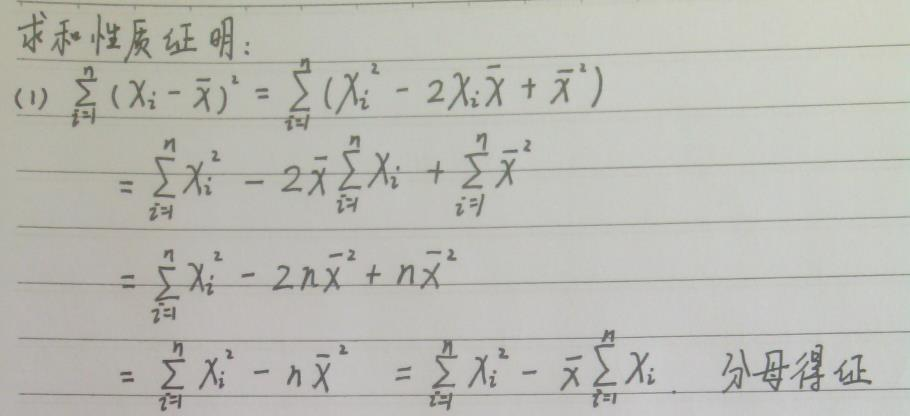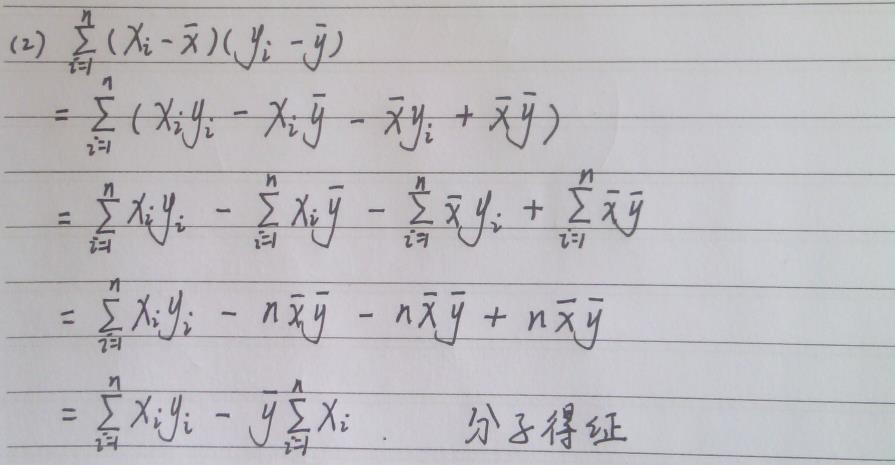一般形式

有了上述推导证明，普通最小二乘法一般形式可以写成（字母盖小帽表示估计值，具体参考应用概率统计）：

y=β1x+β0y=β1x+β0<!--//--><![CDATA[//><!--
y = \beta_1 x + \beta_0
//--><!]]> 的普通最小二乘解为：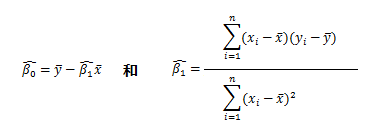多元线性回归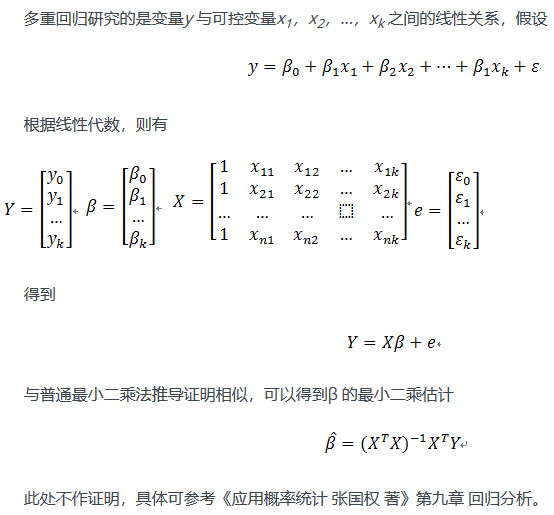最小二乘法和梯度下降法有哪些区别？

最小二乘法的目标：求误差的最小平方和，对应有两种：线性和非线性。线性最小二乘的解是closed-form即 x=(ATA)−1ATbx=(ATA)−1ATb<!--//--><![CDATA[//><!--
x=(A^TA)^{-1}A^Tb
//--><!]]>，而非线性最小二乘没有closed-form（即 (ATA)(ATA)<!--//--><![CDATA[//><!--
(A^TA)
//--><!]]>没有可逆矩阵），通常用迭代法求解。

迭代法，即在每一步update未知量逐渐逼近解，可以用于各种各样的问题（包括最小二乘），比如求的不是误差的最小平方和而是最小立方和。

梯度下降是迭代法的一种，可以用于求解最小二乘问题（线性和非线性都可以）。高斯-牛顿法是另一种经常用于求解非线性最小二乘的迭代法（一定程度上可视为标准非线性最小二乘求解方法）。

还有一种叫做Levenberg-Marquardt的迭代法用于求解非线性最小二乘问题，就结合了梯度下降和高斯-牛顿法。

所以如果把最小二乘看做是优化问题的话，那么梯度下降是求解方法的一种，x=(ATA)−1ATbx=(ATA)−1ATb<!--//--><![CDATA[//><!--
x=(A^TA)^{-1}A^Tb
//--><!]]>是求解线性最小二乘的一种，高斯-牛顿法和Levenberg-Marquardt则能用于求解非线性最小二乘。

莱文贝格－马夸特方法（Levenberg–Marquardt algorithm）能提供数非线性最小化（局部最小）的数值解。此算法能借由执行时修改参数达到结合高斯-牛顿算法以及梯度下降法的优点，并对两者之不足作改善（比如高斯-牛顿算法之反矩阵不存在或是初始值离局部极小值太远）

然后Levenberg-Marquardt方法的好处就是在于可以调节:

如果下降太快，使用较小的λ，使之更接近高斯牛顿法

如果下降太慢，使用较大的λ，使之更接近梯度下降法
展开全文机器学习
• “ OLS”代表“普通最小二乘”，而“ MLE”代表“最大似然估计”。通常，这两个统计术语相互关联。让我们了解普通最小二乘法和最大似然估计之间差异。 普通最小二乘法或OLS也可以称为线性最小二乘。这是一种用于...机器学习
• 本文提出了一种方法，它是将线性模型的参数估计为普通最小二乘回归法，该方法已广泛应用于各种数据集。 最小二乘估计仅考虑了因变量（​​努力）引起误差，而自变量（大小）测量被认为没有误差。研究论文
• 预测精度：线性，普通最小二乘估计将具有低偏差。OLS也表现良好， n >> p。但是，如果 n 不比p大很多 ，则拟合可能会有很多可变性，从而导致拟合过度和/或预测不佳。如果 p > n，则不再有唯一最小二乘...
• 加权最小二乘是对原模型进行加权，是该模型成为 一个新不存在异方差性模型，然后对该新模型使用普通最小二乘法估计参数进行优化。 相关概念梳理： 异方差性解释：随机误差方差不全相等。异方差性是相对于...
• 几种估计办法+例题最小二乘估计（LSE）均方误差（MSE）最大似然估计（MLE）例题 最小二乘估计（LSE） 最合理的参数估计量应该使得模型能最好地拟合样本数据，也就是估计值与观测值之差平方和最小。 注：OLS（普通...
• 比较普遍的参数估计方法： 1、普通最小二乘法：适用于满足经典假设条件但方程模型； 2、加权最小二乘：适合于异方差数据，加权实质是用一个变量除以误差项，使得误差项方差变为常数； 3、工具变量法：适合...
• 为了改进存在复共线性的回归模型中回归系数的最小二乘估计的不足,利用构造岭估计的思想...部分岭估计只修正了很少一部分特征值,所以相对于普通岭估计和广义岭估计的岭参数的确定,部分岭估计的岭参数的确定更加方便精确.
• 算法首先采用非局部均方估计加权最小二乘模型系数，同时用核岭回归作为正则化项进行系数修正，考虑到核岭回归的有偏性，将基于边缘的普通最小二乘模型作为正则化项引进图像插值算法中，并对正则化参数进行自适应调整...
• 统计学分析——基于R ...普通最小二乘估计 fit=lm(y~x) #最小二乘估计 summary(fit) #参数估计，参数检验，回归标准误及参数显著性检验 p 值，决定系数 confint(fit) #参数的区间估计 options(digits=1) ar语言
• 加权最小二乘是对原模型进行加权，是该模型成为 一个新不存在异方差性模型，然后对该新模型使用普通最小二乘法估计参数进行优化。 异方差性解释：随机误差方差不全相等。异方差性是相对于同方差而言...
• 这里向您展示如何在R中使用glmnet包进行岭回归(使用L2正则化线性回归)，并使用模拟来演示其相对于普通最小二乘回归优势。岭回归当回归模型的参数被学习时，岭回归使用L2正则化来加权/惩罚残差。在线性回归背景...
• 是应用最多的参数估计方法，也是从最小二乘原理出发其他估计方法基础。 英语解释：In statistics, ordinary least squares (OLS) or linear least squares is a method for estimating the unknown ...ai python tensorflow 人工智能
• 原始套索算法：原lasso算法在普通最小二乘估计中引入了一个l1-范数惩罚项，如下所示，并使线性回归问题解具有稀疏性，调整正则化参数λ以控制解稀疏度。 本算法创新点： 1、 用(负)对数似然函数替换最小二乘...
• 在这种情况下，模型或数据的微小变化就可能导致多元回归模型的系数估计值出现不规律地改变，可能造成如下后果：回归系数的普通最小二乘估计量可靠度降低。如图1与2所示，随着多重共线性程度的提高，参数方差（ 表示...
• 在这种情况下，模型或数据的微小变化就可能导致多元回归模型的系数估计值出现不规律地改变，可能造成如下后果：回归系数的普通最小二乘估计量可靠度降低。如图1与2所示，随着多重共线性程度的提高，参数方差( 表示...
• 普通最小二乘话计相比，岭估计可以降低参数估计的均方误差，但是却增大残差平方和，拟合效果变差。本文提出一种基于泛岭估计对岭估计过度压缩改进方法，可以改进峰估计的拟合效果，减小岭估计残差平方和增加...机器学习
• 第七章岭回归1.岭回归估计是在什么情况下提出？答：当解释变量间出现严重多重共线性时，用普通最小二乘法估计模型参数，往往参数估计方差太大，使普通最小二乘法...答：一种改进最小二乘估计的方法叫做岭估计。...
• DolphinDB特色 DolphinDB集成高性能数据库和功能齐全脚本语言。 集成一个优点，是我们可以直接在数据库中分析数据。 直接在SQL语句中调用函数ols ...返回对X和Y计算普通最小二乘回归结果。 o...DolphinDB sql
• 在这种情况下，普通的最小二乘估计量会受到严重偏见，这也受到审查输出和噪声输入影响。 为了解决该问题，我们开发了一种有效偏差补偿Heckman算法（BC-Heckman），用于带有噪声输入删失回归。 BC-Heckman...
• 岭回归是一种专用于共线性数据分析有偏估计回归方法，实质上是一种改良的最小二乘估计法。通过放弃最小二乘法无偏性，以损失部分信息、降低精度为代价获得回归系数更为实际和可靠回归方法，对病态数据拟合能力...
• 这里向您展示如何在R中使用glmnet包进行岭回归（使用L2正则化线性回归），并使用模拟来演示其相对于普通最小二乘回归优势。 岭回归 当回归模型的参数被学习时，岭回归使用L2正则化来加权/惩罚残差。在线性回归...
• ## 统计模型检验

千次阅读 2011-10-04 20:47:24
文如其名，该文对不同统计模型检验进行了概括，其中包括普通最小二乘回归（OLS）、分位数回归（QR）、稳固回归（RR）、嵌套对数模型（NLM）、泊松及负二项模型(PANBM)、非参和半参数估计（NASE）、偏最小二乘（PLS...estimate regression